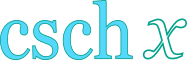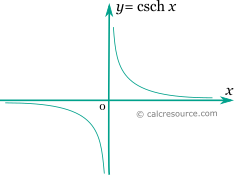Hyperbolic Cosecant Calculator

This tool evaluates the hyperbolic cosecant of a number: csch(x). Enter the argument x below.

 x = Result: csch(x) =Definitions

General

The hyperbolic cosecant function is defined as:

The graph of the hyperbolic cosecant function is shown in the figure below.Series

All hyperbolic functions can be defined in an infinite series form. Hyperbolic cosecant function can be written as:

The above series converges for . Bndenotes the n-th Bernulli number.

Properties

The derivative of the hyperbolic cosecant function is:

The integral of the hyperbolic cosecant is given by: